1.10 Probability

Introduction: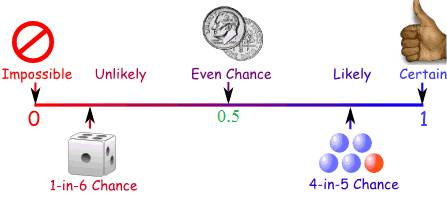The above picture summarises  chances of some thing happening from 'impossible' to 'possible'.

As we know ‘probability’ means chance of some thing happening. It could be any of the following:

1.    The chances of rains on any particular day

2.    The chances of India winning cricket test matches, one-day world cup

3.    A cricketer scoring century in a particular test match

4.    India winning Olympic medals in 400 meters relay

5.    Number of times  head comes up when  1 rupee coin is tossed  up 100 times

6.    Number of times  number 2 appears when a dice is drawn 500 times

The outcome of  any of the above event is pure chances. It may happen or it may not happen. Do you notice the difference between   first 4 statements and the last 2 statements? In case of first 4 cases, the result depends upon external factors such as place, time, strength of opposition team.. where as result of 5 and 6  will almost be same irrespective of place and time of the event.  There are cases when outcome of certain events can be forecast with some certainty. One such event is tossing of coin. When we toss the coin there can only be 2 outcomes, either head appears or tail appears. When coin is tossed up several times obviously, the chances of head coming up can vary from 0% to 100% and tail coming up can vary from 100% to 0%. However when  coin is tossed up in large number of times(in thousands)  head coming up may be 50% and tail coming up could be 50%.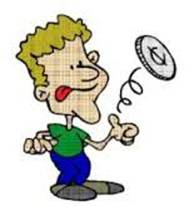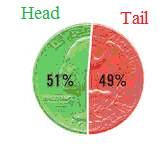In such cases, the probability is 1/2. Note that when head appears tail can not appear at the same time. Similarly when tail appears, head can not appear at the same time. This  we say that events are exclusive. That is to say, there can not be any other possibility other than head appearing or tail appearing. In such case, we note that the sum of their probability is 1/2+1/2= 1. Note that probability is expressed as a ratio and it is always ≤  1. why does dice have dots numbers from 1 to 6?.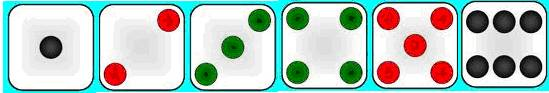Note that dice is a cube and it has 6 faces. Hence their faces are represented by 1 to 6 dots representing numbers from 1 to 6. When a dice is cast what are the chances of a number appearing from 1 to 6? It is one out of 6 and hence the probability is 1/6.

What is the sum of probabilities of number appearing between 1 and 6?

It is = Probability of number 1 appearing+ Probability of number 2 appearing + Probability of number 3 appearing + Probability of number 4 appearing + Probability of number 5 appearing + Probability of number  appearing between 1 and 6

= 1/6+1/6+1/6+1/6+1/6+1/6= 1

(Note that, when one number appears when a die is cast, other number can not appear at the same time. Since probability of each number appearing is excusive of others, sum of their probabilities is equal to 1.

Probability of number 1 not appearing

= Probability of number 2 appearing + Probability of number 3 appearing + Probability of number 4 appearing + Probability of number 5 appearing + Probability of number 6 appearing = 1/6+1/6+1/6+1/6+1/6= 5/6

This also can be calculated as

Probability of number 1 not appearing = 1 - Probability of number 1 appearing = 1-1/6= 5/6

Let us learn some of the definitions of terms used

 No Term Meaning Examples 1 Experiment Test or a procedure or operation which produces a result. In this lesson all experiments will be random. 2 Trial Performing an experiment tossing a coin/throwing a dice 3 outcome Result of trial head or tail/ appearance of number from 1 to 6. 4 Sample space Set of all possible outcomes S = {H,T}. S = {1,2,3,4,5,6} Event Every  subset of  Sample Space Getting the head=A= {H}, Getting the number 4=B={4} Getting even numbers= C = {2,4,6} 5 Elementary event Each of the outcome in sample space. That is  each of the element in sample space. (Every subset of sample space having only one element) Getting the head=A= {H}, Getting the number 4=B={4} 6 Compound event Obtained by combining one or more elementary events ( Subsets of sample space having one or more element) Getting all three heads A= {HHH} Getting at least 2 tails B = {HTT, TTH, THT, TTT} 7 Favourable event An elementary element that occurs 8 Certain(sure) event If any one of the event will occur in the trial (probability of sure event is 1) When a die is cast the probability of getting a number > 0 and <  7 is 1. (Because when the die is cast the numbers got are  always between 1 and 6). 9 Impossible event An event which will not occur in any of the trial (Probability of impossible event is 0) when a die is cast the probability of getting number <1 or >6 is 0.(Because this event will not occur at all). 10 Complementary event Converse of elementary event. if element A is denoted by A then complementary event is denoted by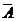.  Note that A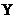= S and A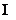=  {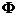} Getting head A = {H}, converse of this is not getting head that is getting tail. Hence= {T} Getting the number 4, B={4}, converse of this is not getting 4, that is getting numbers 1,2,3,5 and 6. Hence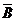= {1,2,3,5,6} 11 Mutually exclusive event 2 or more events are said to be mutually exclusive if those events do not occur simultaneously, that is they do not happen together Let S = {1,2,3,4,5,6},  A = {1,2,3},  B = {4,5} Here A and B are mutually exclusive, because either A occurs or B occurs. they do not occur at the same time. Also note that  AB  =Experiment Sample space and Events No of events n(S) Event A Favourable outcomes to event A and n(A) Probability P(A) = n(A)/n(S) Complementary Event( not A=) Probability of Complementary Event P() = n()/n(S) 1 coin is tossed S={ H,T } 2 Tail coming up A=(T) n(A)=1 1/2 head comes up= (H) n()=1 1//2 2 coins are tossed S={ HH,HT,TH,TT } 2*2=4 Tail should not come up A = {(HH)} n(A)=1 1/4 Tail comes up n()=3 3/4 3 coins are tossed S={ HHH,HHT,HTH,THH, HTT,           THT,TTH,TTT } 2*2*2=8 Same face coming up A= {(HHH),(TTT)} n(A)= 2 2/8 Not all same faces n()=6 6/8 1 dice is cast S={1,2,3,4,5,6 } 6 Even number coming up A={2,4,6} n(A)= 3 3/6 Odd number coming up n()=3 3/6 2 dices are cast S={(1,1),(1,2),(1,3),(1,4),(1,5),(1,6)         (2,1),(2,2),(2,3),(2,4),(2,5),(2,6)         (3,1),(3,2),(3,3),(3,4),(3,5),(3,6)         (4,1),(4,2),(4,3),(4,4),(4,5),(4,6)         (5,1),(5,2),(5,3),(5,4),(5,5),(5,6)         (6,1),(6,2),(6,3),(6,4),(6,5),(6,6)        } 6*6=36 Getting  the same number A= {(1,1),(2,2),(3,3), (4,4),(5,5),(6,6) n(A)= 6 6/36 Different numbers n()= 30 30/36

P(E) = Probability of an event PE =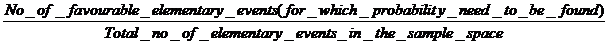=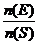Observe that P(A)+ P() =1  (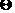n(A)/n(S) + n()/n(S) ={ n(A) + n()}/n(S) = n(S)/ n(S) )P(A) =1- P(), P() =1- P(A)

Probability: It is the ratio of the number of elementary elements favourable to the event E to the total number of elements in the sample space

Can probability ratio be 0 or 1?

1.    What is the probability of getting a number 0  or greater than 6 when dice is cast?

In this case  A = {} an empty set,  and n(S) = 6  , hence P(A)= n(A)/n(S)= 0 (When a  dice is cast number is  one among 1 to 6 only). This we call an impossible event, because such a event does not happen

2.    What is the probability of getting a number  1 to 6 when dice is cast?

In this case  A = S   and hence n(A) = n(S) = 6  , Thus P(A)= n(A)/n(S)= 1 (When a  dice is cast number is always  one among 1 to 6). This we call a sure  event, because such a event always    happens

Thus 0     P(A) ≤  1 This is what we represented in the beginning by a figure: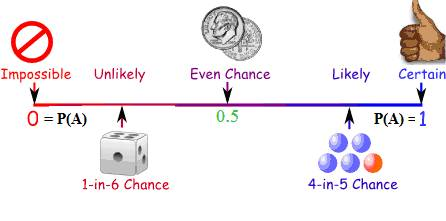Problem 1 : A game consists of rolling 2 dices. If the sum is 2,3,4,5,10,11 or 12, player A wins. If sum is other than these, then player B wins. Of you want to win, would you be player A or player B?

Solution:

Let A = { combination of dices whose sum is 2,3,4,5,10,11 or 12  }= { (1,1),(1,2),(2,1),(1,3),(3,1),(2,2),(1,4),(4,1),(2,3),(3,2),(4,6),(6,4),(5,5),(5,6),(6,5),(6,6) }

Then B=={ combination of dices whose sum is not 2,3,4,5,10,11 or 12 }= { combination of dices whose sum is 6,7,8, or 9 }

= {(1,5),(5,1),(2,4),(4,2),(3,3),(1,6)(6,1),(2,5),(5,2),(3,4),(4,3),(2,6),(6,2),(3,5),(5,3),(4,4),(3,6),(6,3),(4,5),(5,4)}

Note that n(A)= 16 and n() = 20. Thus number of elementary elements= 36( Other wise also note 6*6=36: fundamental principle of computing)

P(A) = 16/36 = 0.4444 and P()= 20/36= 0.55555

Obviously Any one would like to be B.

Mutually exclusive event

Let S = {A1, A2, A3 …. An} Clearly n(S)= n, P({A1})= 1/n , P({A2})=1/n, P({A3})=1/n … P({An})= 1/n

We note that, P({A1})+ P({A2})+ P({A3})+ . . . P({An})= 1/n+1/n+1/n + . . . 1/n= n/n= 1

So, sum of the probabilities of all the elementary events of an experiment is 1.

Let E1 and E2 be two mutually exclusive elements, that is they will not have any common elements. We have observed that E1E2  = {}

They being disjoint sets, from set theory, we know  n(E1E2)  =  n(E1)+n(E2)n(E1E2)/n(S)= n(E1)/ n(S)+n(E2)/ n(S)P(E1E2) = P(E1)+P(E2)

In general if E1, E2, E3 . . . En  are mutually exclusive elements  then P(E1E2E3 . . .En ) = P(E1) +P(E2)+ P(E3)+ . . . P(En)

Problem 2 : When a die is thrown, find the probability that either an odd number or square number occurs

Solution:

S= {1,2,3,4,5,6}, n(S)=6

A= { Odd numbers or square numbers} = {1,3,4,5}, n(A)=4P(A) = 4/6

Problem 3 : The outcome of a random experiment results in either success or failure. If the probability of success is thrice the probability of failure, find the probability of success

Solution:

P(A) =Probability of success, Probability of failure= P()

P(A) = 3P() =3x, we also know that P(A)+P() = 1;P(A)+ 1/3P(A)=1

4P(A)= 3  P(A)= 3/4

Problem 4: Three squares of a chess board are selected in random. Find the probability of getting two squares of one colour and the other of different colour.(here square represents smallest square on which chess is played)

Solution:

Note that chess board has 64 smallest squares.

3 squares can be selected in 64C3  ways. Hence n(S)= 64C3 =64*63*62*61!/61!*3!= 64*63*62/2*3= 64*21*31

Number Of black coloured squares = 32, Number of white coloured squares=32

2 squares can be got in 32C2  ways. 1 square can be got in 32C1 ways.

If A is number of ways getting two squares of one colour and the other of different colour, then n(A)= [{32C2}*{32C1}]=[{32*31/2!)}]*{32}=32*31*16P(A) = 32C2*32C1/64C3= 32*31*16/64*21*31=8/21

Problem 5 : A committee of 5 persons is selected from 4 men and 3 women. What is the probability that the committee will have

(i)  one man (ii) 2 men (iii) 2 women (iv) at least 2 men

Solution:

Note that total number of people  available to form the committee is 7.

Hence number of ways of forming different 5 member committees = 7C5= 7*6/2!= 21= n(S)

(i) one man in the committee:

Since there are only 3 women, we will need 2 men to form a committee of 5. Hence, the probability of  forming a committee  having  one man is 0

(ii) 2 men in the committee:

Number of ways committees that can be formed with 2 men from 4 men =4C2= 4*3/2!= 6

Remaining  3 members are to be women.

Number of ways committees that can  be formed with 3 women  from 3 women =3C3= 1The number of committees that can be formed with 2 men and 3 women = 6*1=6            ---------(1)Probability = 6/21= 2/7

(iii) 2 women in the committee:

Number of committees that can be formed with 2 women from 3 women = 3C2= 3/1!= 3

Remaining 3  members are to be men.

Number of ways committees that can  be formed with 3 men from 4 men =4C3=4/1!=  4The number of committees that can be formed with 2 women and 3 men = 3*4=12         -----------(2)Probability = 12/21= 4/7

(iv) at least 2 men in the committee:

Number of committees that can be formed with at least 2 men

= Number of committees that can be formed with 2 men+ Number of committees that can be formed with 3 men+ Number of committees that can be formed with 4 men

First we need to find  number of committees that can be formed with 4 men.

Number of committees that can be formed with 4 men from 4 men = 4C4= 1

Remaining 1  member has to women.

Number of ways committees that can  be formed with 1 woman from 3 women =3C1=3*2!/2!*1!=  3The number of committees that can be formed with 1 woman and 4 men = 1*3=3  ------------------(3)Number of committees that can be formed with at least 2 men = 6+12+3 =21Probability = 21/21= 1

Problem 6 : As shown in the adjoining figure a dice has been cast in the rectangular shaped area. What is the probability of die falling inside the circle of diameter 1m inside the rectangular area?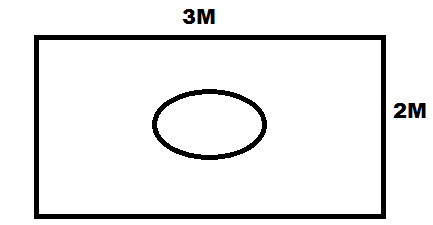Solution:

Area  of rectangular area= ಆಯತದ ವಿಸ್ತೀರ್ಣ =6  sq.m.

Area of circle =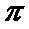*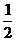*sq.m..(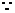Radius isಮೀ. )

Probability of dice falling inside circle   = Area of circle÷ Area of rectangle =**÷ 6=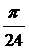1.1 Summary of learning

 No Points studied 1 Probability,Event,Favourable event, exclusive Event